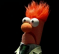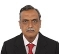## Bit error rate (bit off topic)Started by 4 years ago11 replieslatest reply 4 years ago103 views

Hi everyone,

Sorry for this bit off-topic question, but maybe someone can help. I'm pretty sure the answer is simple, but I don't seem to be able to google the right question.

I have done a test in my embedded system to detect the bit error rate. However, after checking 2855176 bits, none of them flipped. What is the upper limit of the BER and with what confidence?  How is this called in statistics? Any help would be appreciated!

Thanks!
Vincent

[ - ]The key phrase is "confidence interval". If a search on that doesn't bring joy, check back here.
[ - ]Thanks Tim!

You give me something to read.  Gee, is my statistics rusty!

Regards,
Vincent

[ - ]So, I looked at confidence interval, but if I enter the values into the specified formula, I end up with nothing. This is because I never encountered a bit-flip. I think the formula for calculating the BER upper limit for a specific confidence C of not seeing a bit flip should be something along the lines of

C = 1-(1-b)^N

Where N is the number of samples which you did *not* see a flip, and b the unknown BER. E.g. for a BER of 0.01 and a population of a 100, the chances of seeing bit flip would be 63%.

Doing some manipulation to get b out of the equation, I come to the following:

b = 1-E^(log(1-c)/N)

Plugging in the numbers 1-E^(log(1-0.95)/2855176) = 4.55e-7

As the with 95% confidence the BER is 4.55-7 or lower. As verification, if I plug in the example with N=100 and C=0.63, I get 0.01.

The question is, what did I calculate :)

[edit: I think I am close, but I messed up seeing and not seeing a bit flip]

[ - ]I think that $$b = 1 - e^{\frac{\log(1-C)}{N}}$$ is correct, but there's something wonky about the result you got when you plugged in numbers -- maybe you used $$\log_{10}$$ instead of the natural log?

At any rate, for $$a \ll 1$$, $$1 - e^{-a} \simeq a$$, so you can simplify your computation considerably.  I get a BER a bit more than $$1 \cdot 10^{-6}$$, which seems more reasonable for that confidence level -- your calculated BER is pretty close to $$\frac{1}{N}$$, which doesn't scan for me.

[ - ]How do you write math code in here?

Anyway, you're right. I assumed to the log was actually the natural log, but it wasn't. Anyway, is $a = ln(1-C)/N$ ?  (trying Latex code here)?

For your other question about sensibility  I can't disclose too much, but this concerns an RF technology. It is more that I want to measure the fault rate in the receiver. Forward error correction (4/5) is applied, but this is not very reliable. So, this complicates things a bit, as wrongly applied error correction may yield multiple bits flipped. But for now I wanted to keep things simple.

So strictly speaking it is not really a bit error rate in the traditional sense, sorry about that, but I did want to measure the chance of one or more bits flipping.

I did not find any faults, but I still want to put some figure on this. The test was about 4 days, but not all the time, it is hard to put a figure on it.The cost of a bit flip will be that losing a packet of data, as the packet is verified with a 32 bit checksum after this point.

[ - ]The forum uses MathJax to embed LaTeX code.

You can use the LaTeX construct \ ( <math code here> \ ) for inline, and \ [ <lots of math code here> \ ] for stand-alone.  Just take the spaces out between the slashes and the things following, and (as long as you're facile with LaTeX) you should be fine.

[ - ]Just for sensibility:

• How long did you do the test for?
• What's the cost of a bit error?
• What recovery mechanism do you have for a bit error?
• What's the BER after someone has tripped over a cable and yanked it out of your machine, or cut it with a backhoe, or done whatever real-world violence is going to happen to it?
[ - ]As per my understanding the BER is explained below:

What is Bit error rate?

Bit error rate: the error rate to which the communication system allows that it can have a sustained communication.

How it is defined?

1 bit error in n number of bits communicated. For example if it is defined as BER = 10^(-6), then there can be 1 error in 10^6 bits communicated.

How it is measured?

It is measured in the number of packet losses in the communication channel. Also it is assumed here that packet loss is due to 1 bit error, but it can be more.

How error is detected?

In order to detect the error, the packets will be defined with checksum or CRC, the method with which the integrity of the data is confirmed. This will be part of the packet and will not be part of the data. At transmission end, the checksum is calculated and appended to the packet and at receive end it is calculated and compared for the integrity of the packet.

At transmission end mostly it is assumed that it is error free, (however there are some systems which will keep track with local echo and confirms it in some of systems).

At the receiver end, the received packet is analyzed. The received packet may be full, or may be a part of packet and receiver will abandon the packet by timing out. Once full packet is received, if it fails in checksum test it flags the received packet is erroneous.

How error rate it is computed?

The number of bits of received data and transmitted data can be accumulated and at each time of there is an error in the packet, the error count is incremented. Also at receiver end if there is a partial packet is received, and terminated due to time out, the received number of bits are counted and the ratio of no of errors to total number of bits will give bit error rate.

In computation the total number of bits is both bits transmitted and bits received. Since transmitter assumes all has gone well, it accumulates transmitted bits as it is and accumulates the actual received bits.

This has to be done at both ends. Finally during computation received bits at both ends to be considered.

Example:

Let us consider a RS232 communication channel working at 115.2 kbps. Let us consider that it is working at 8N1, (8 bits no parity 1 stop bit). So 1 byte will be transmitted as 10 bits, and 1 packet of data (which may vary depending on the size of packet) will have n*10 bits.

Assume that packet will be of 100 bytes on average for data packets, 16 bytes for command packets and 8 bytes for Ack or Nak packets.

If there are 100 packets communicated with ~ 30 data packets 40 command packets and 24 Ack and 6 NaK pckets and there are 4 packet error, then

Total communicated bits = 30 data = 30*100*10 = 30000 bits,

40 command = 40*16*10 = 6400 bits,

24 Ack = 24*8*10 = 1920 bits,

6 Nak = 6*8*10 = 480 bits,

= 38800 bits.

Error = 4,

Hence BER = 4/38800 = 1.031E-4

Hope this will give some amount of clarity.

Best Regards,

BV Ramesh.

[ - ]BER for what particular device or communication scheme or ?? How are you testing and measuring?

I spent many many many hours doing BER testing for various RF digital communication schemes during college and early career. It can take a very very long time to measure a low BER. For example, if the expected BER is 10^(-12), you might have to test 10^14 bits before seeing an error... (something like that... not sure if I'm remembering the exact rule of thumb here..)

Best regards,

Matthew

[ - ]You might take a look at the Wikipedia page on bit error rate, a good starting point for many topics.

I don't know anything about your transmission environment, so I'll assume for simplicity that you have some manner of typical bit serial transmission. You're sending a bit sequence, and the receiver is sampling each bit of data. If the sampling clock relative to data setup and hold time is fat with timing margin, your MTBF is going to be a lifetime of the universe kind of number and your BER is so close to 0 that you don't care about the difference.

3 Mbits is not much data. Unless your transmission system is really poor with a relatively high probability of bit errors, it isn't too surprising that you haven't seen an error.

Things get much more interesting when you crank the data rate way up, use sophisticated multi-level encoding schemes, and recover timing information on the receive side.

Here is a pointer to a Keysight article (I don't represent them or have any skin in their game) regarding bit error rate measurement and confidence level that you may find interesting.

[ - ]Thanks for all your replies. The test has ended, and I can not take any more data at this time. It was a slow radio communication channel.

So the question is: can I say anything at all about the upper limit of the BER, without actually finding any bitflips. Eg. At least BER <= 1/2855176 with 100% confidence (which is not the right answer, I know)

So, in my mind I think I can simplify it to the following mind-game:

* having a dice with N faces, of which all are 0 (no flip) except one 1 (flip).

* Question: What is the chance of throwing 2855176 zeroes with a specific dice of N faces 95% of the time (95% confidence)?

* The dice with size N that gives me this result would be the upper limit of the BER with 95% confidence, right?

Anyway, as I said, I'm trying to get my mind around it.